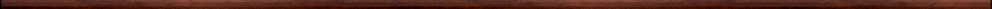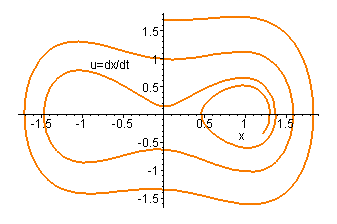Maple worksheets on the Duffing equationNumerical methods topics:

They are all compatible with Classic Worksheet Maple 10.Introduction and free oscillations - duff1.mws

• The double-well oscillator and the Duffing equation
• A periodic solution through both wells

Damped oscillations - duff2.mws

• The Duffing equation for damped oscillations
• A sample numerical solution
• The two basins of attraction in the phase plane
• Solutions attracted to each well

Forced and damped oscillations - duff3.mws

• The Duffing equation with both damping and forcing terms
• A non-chaotic solution
• The transition to chaos

Forced and damped oscillations (continued) - duff4.mws

• A periodic solution with period 4 times the period of the driving force
• Reconstructing the solution and its symmetrical counterpart
• Poincare sectionsTop of page

Main index